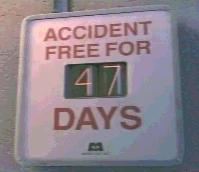# 47

This number is a prime.

Just showing those entries submitted by 'Gaydos': (Click here to show all)© Disney/PixarPrime numbers that end with "47" occur more often than any other 2-digit ending among the first one billion primes. [Gaydos]The sum of the first 47 positive integers with digits in strictly ascending order is divisible by 47 (2350/47=50). The next and largest such prime is 383 (1848741/383=4827). [Gaydos]47 (exactly half) of the first 94 prime numbers each has at least one prime twin. [Gaydos]47 squares are each adjacent to at least one distinct digit prime number. [Gaydos]The sum of the first 47 positive palindromic numbers is a palindrome. [Gaydos]

(There are 9 curios for this number that have not yet been approved by an editor.)